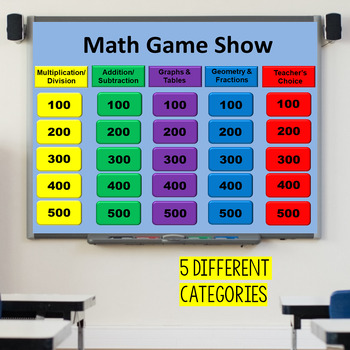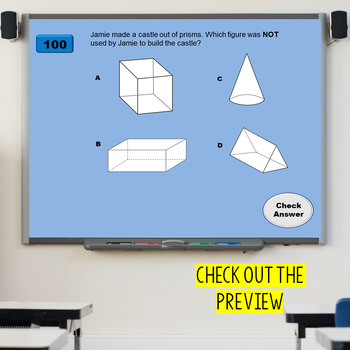# Math Review Game 3rd Grade Test PrepGrade Levels
3rd
Subjects
Standards
Resource Type
Formats Included
• PPTX
Pages
54 pages

### Description

This is a 3rd grade test prep review game. It was made to review the 3rd grade Math STAAR test but can be used with any state assessment.

This game is like a "Jeopardy" style game. The categories include multiplication/division, addition/subtraction, graphs, generate/extend tables, and teacher's choice. The questions are all word problems that get more difficult as you increase in value.

An example of a question is listed.

Janelle has 7 pages of stickers that have 12 stickers each. Katy has 8 pages of stickers that has 9 stickers each. How many more stickers does Janelle have than Katy?

The template is editable if you need to change the questions.

TEKS addressed:

3.4A, 3.4B, 3.4D, 3.4H, 3.4K, 3.5B, 3.5C, 3.5E, 3.8A, 3.8B

Superhero graphics are provided by awaywiththepixels.

****************************************************************************

My Other Related Product:

Game Show Template

****************************************************************************

How to get your TPT credit to use on future purchases:

Make sure to provide feedback on every item you buy. Click on the Provide Feedback button and give a rating and comment about the product you purchased. TPT gives a credit that you can use to lower the cost of future purchases.

*Look for the green star near the top of any page within my store and click it to become a follower. You will then receive email updates about this store.

I hope you enjoy this product!

Thanks,

Jennifer

Total Pages
54 pages
Answer Key
Included
Teaching Duration
N/A
Report this Resource to TpT
Reported resources will be reviewed by our team. Report this resource to let us know if this resource violates TpT’s content guidelines.

### Standards

to see state-specific standards (only available in the US).
Identify arithmetic patterns (including patterns in the addition table or multiplication table), and explain them using properties of operations. For example, observe that 4 times a number is always even, and explain why 4 times a number can be decomposed into two equal addends.
Solve two-step word problems using the four operations. Represent these problems using equations with a letter standing for the unknown quantity. Assess the reasonableness of answers using mental computation and estimation strategies including rounding.
Understand division as an unknown-factor problem. For example, find 32 ÷ 8 by finding the number that makes 32 when multiplied by 8.
Apply properties of operations as strategies to multiply and divide. Examples: If 6 × 4 = 24 is known, then 4 × 6 = 24 is also known. (Commutative property of multiplication.) 3 × 5 × 2 can be found by 3 × 5 = 15, then 15 × 2 = 30, or by 5 × 2 = 10, then 3 × 10 = 30. (Associative property of multiplication.) Knowing that 8 × 5 = 40 and 8 × 2 = 16, one can find 8 × 7 as 8 × (5 + 2) = (8 × 5) + (8 × 2) = 40 + 16 = 56. (Distributive property.)
Determine the unknown whole number in a multiplication or division equation relating three whole numbers. For example, determine the unknown number that makes the equation true in each of the equations 8 × ? = 48, 5 = __ ÷ 3, 6 × 6 = ?.

### Questions & Answers

Teachers Pay Teachers is an online marketplace where teachers buy and sell original educational materials.

More About Us